Math Modeling : Chaos and Fractals

Example Questions

Example Question #1 : Dynamic Models

There are two similar species of monkey that inhabit the same forest. The intrinsic growth rate is 4% for species A and 7% for species B. The capacity of the forest is estimated at 125,000 specie A monkeys and 450,000 species B monkeys. Poaching these monkeys over the last 100 years has caused for a decline in each species. Species A has a population of 4,000 and species B, a population of 80,000. Use a discrete model to project the population growth with a time step of several years. How large a time step can be used to maintain a continuous time model with an equivalent qualitative behavior?

Assume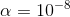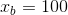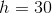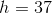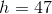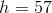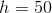Explanation:

Identify the known information and the dynamical system.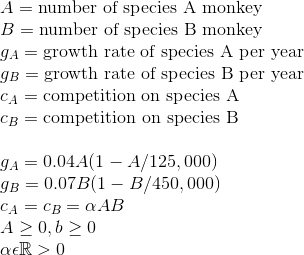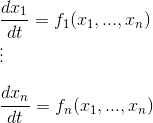The dynamical system equation is: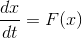From here solve by using the Euler Method for a discrete time dynamical system.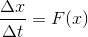Now formulate the model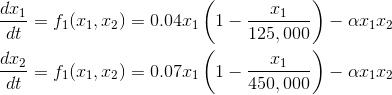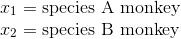Now transform these equations into a set of difference equations.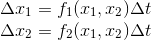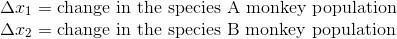Assume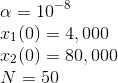whererepresents the number of iterations the computer will use when implementing the Euler Method.

The time step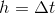will need to be changed and the model reformulated to calculate how large the time step can be before it doesn't resemble a continuous time model.

Using computer implementation of the Euler Method on the population of species B monkeys the following graph is produced.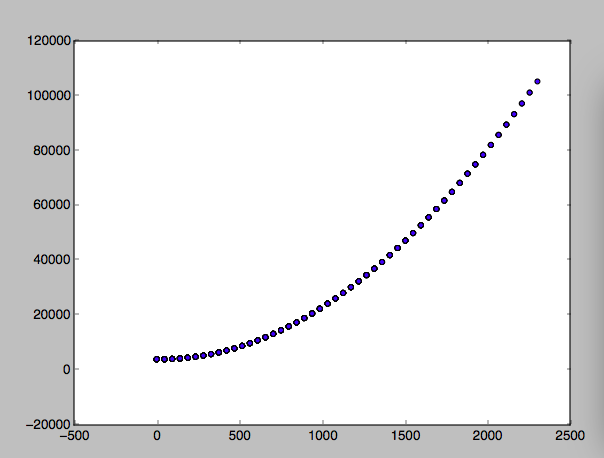It is seen thatis the largest time step before the function begins to have chaos and not represent the behavior of a continuous system.